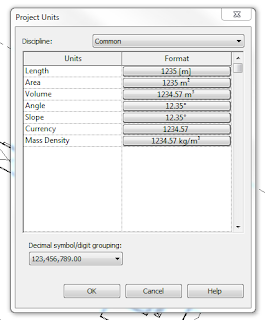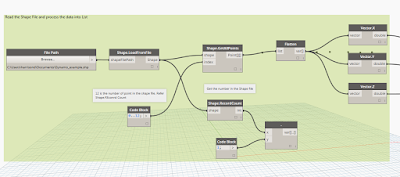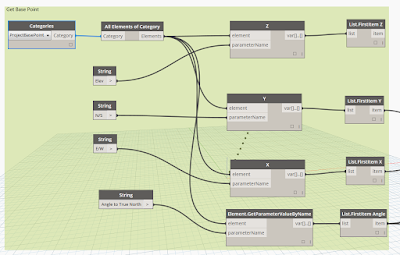InfraBIM - Infrastructure BIM & GIS

BIM works flows of using Autodesk products, such as Infraworks, Civil 3d, Map 3d, Recap Pro and Navisworks that makes my life easier.

Thursday, January 12, 2017

BIMFix Framework for Shared Model Establishment

BIMFix Framework for Shared Model Establishment_v1-0
Brian Renehan has a blog , BIMFix has produced an excellent workflow for establishing shared coordinates in Revit and exchanging data between software platforms.
For Building Lead Consultants using Autodesk® Revit®
This free downloadable framework is a document which can be referenced from or attached to a BIM Execution Plan (BEP). The Framework provides the Project Team clear methodologies on how to establish and share Project BIM (Building Information Modeling) Models, where the lead design consultant is using Autodesk® Revit® as their primary authoring application. The framework is designed to be flexible in meeting project needs, work across BIM/CAD software platforms, and for actions to be controlled and traceable.
The detail can be found on BIMFix Blog

Friday, May 27, 2016

Walk to Work.

I walk to work every day from the train station to the office. I thought I'd  share my journey, with the entire route which has been captured by point cloud and all the in ground services modelled.
The 1.5 km Journey does not take me 2:30 minutes
The point cloud data equated to 3 GB per 100 meters. The point cloud data was processed with Recap Pro and service modelled in Autodesk Civil 3d. Both data sets were overlaid in Autodesk Navisworks and the viewport transition to create the animation.
So how did I manage to Create a render animation with 3 GB x 15 = 45GB.
The workflow to create an animation with s large point cloud data set
1. Create and save the first viewport. The viewport needs to have the correct target eye level.
2. Apply a section box to the view, while keeping in mind the view depth to be limited. The intent of the section is to limit the amount of point per view when rendering the animation.
3. Update the viewport. Edit the viewport setting.
4. Pan forwards 100m or so to the desired location. Save the viewport and drag the section boxes.
5. Keep creating and staging the viewports, until the final desired location.
6. Now to stitch all the views together, create animation folder and drag in your views.
7. Click play and watch your animation.
.

Tuesday, April 12, 2016

Importing Revit files into Infraworks

Importing your Revit model into Infraworks is a simple workflow and can be done in 3 ways.

1. Native Revit file imported via the cloud processing tool in Infraworks / or the local processing tool.
2. Exporting Revit via FBX (Revit always exports FBX units as feet) and importing FBX into Infraworks.
3. Export Revit via DWG (metric Revit files export as metric. Great for massing options) and importing into Infraworks.

My preferred workflow is using FBX, why this method?

• Exporting is done by FBX as it is sent to from Revit to Infraworks. Infraworks does allow RVT files (via the cloud / or local processing if you have Navisworks Manage installed) to be imported but I found that if you can create an FBX file (with LOD used) of the exact model you require. Ie turning off all internal elements (furniture) in a saved 3d view making a smaller FBX file (hence a smaller AIM model). Similar workflow when exporting Navisworks from Revit, controlling the output.

• The Basepoint in Revit is the model setout point of the exported FBX. You need to record the coordinates of the Basepoint as this will be used to position the FBX file in the correct location. This is based on the fact that you have set the model up with the correct coordinate which related to the site which is known the coordinate system.

The above image of the Revit model showing that the model has being rotated to True North and that the base point is located in the PCG94 grid system.Import FBX file which has not been configured
The known coordinates system is inputted. Ie PCG94 in this example.
The x been the E/W from Revit 53800.6077, The Y been the N/S from Revit 264989.7382 and the Z been the Elev from Revit 17. Before recording the values, I change the Revit unit to Meter.FBX location define in the configuration
Before recording the values, I change the Revit unit to Meter.Revit Units Set to Meters
• The Result of the building FBX file is imported into Infrawork using the Base point as the setout for the file. The model appears in the correct location but incorrectly rotated. What was not done during the configuration of the FBX file was input the True North rotation from Revit base point

• The strange part to get the model to be correctly rotated is to take the Revit rotation to true north and minus that from 360. Eg. 360°- 207.332°  is = to 152.668. using the value you input that into the configuration of the rotation for the Z.The Z value of the Revit ration to True North - 360.
The short video below is a workflow that I've used to export Revit to FBX which then imported into Infraworks.

Saturday, February 27, 2016

InfraBIM Dynamo Nodes

A brief explanation of my custom nodes.

Transform_in I use this node when bringing world coordinates data into Revit. The node takes the project define base point and true north angle and 2d matrix transforms and subtracts against the input values. The result been the value are now relative to project base point. ( aka smaller.)

Transform out.
I use this node when getting world coordinates values out of Revit. The node takes the project define base point and true north angle and 2d matrix transform and adds to the elements local coordinate values, resulting in world coordinates.

XYZ parameter. This is not so much as a custom node but more of a time saver. I have reparations uses of this node when inputting or modifying elements with xyz parameter and world coordinates parameters. Formwork Area I use this node to get the split surface areas based on the direction of Z value relative to the surface. The result, the Top, Bottom and side area values This is not so much as a custom node but more of a time saver. I have reparations uses of this node when inputting or modifying elements with xyz parameter and world coordinates parameters. Basepoint. This node is used to input the project base point. Example of use is when creating topographic surface from a landxml file. The process of trash and burn.

Tuesday, January 26, 2016

GIS into Revit using Dynamo

Revit does not have a import function for a GIS ESRI Shapefile. There are multiple work follows of using MAP 3d or Civil 3d and then importing a .dwg into Revit. Dynamo and the package of GIS  now allows the import function of points, curves and Polygons. The current Package on Dynamo only imports the geometry with in the Shape file and not attributed information, this is currently not available with in the GIS package.

The image of the Dynamo script below uses GIS package in Dynamo to read the shape file and insert point families into Revit. As noted before only the point and curve geometry is import into Revit, no attributed data. The Points are matrix transposes the point to suit the Revit base point to insert point base family in the correct location.The above appears to be complex set of node but real are just a simple connection of node that performs a simple task;

1. Read the ESRI Shapefile
2. Process the ESRI Shapefile into list
3. Get the Project Base Point and rotation
4. Matrix transform the point form the Project base point
5. Insert Point family from the transformed base pointsThe above image is a group of nodes to read the ESRI Shapefile

The process in the above image includes to read the entries of the points with in the Shape file. The result of the list on points is need to be inserted into the Code block to list

Group of nodes to get the project base Point.The next node group is thanks to Wikipedia! If you can under stand Revit shared coordinates well try think of it backwards!!!

Revit's project base point is the mathematical set out point of the graphical data base. The project base point removes large numbers from the data base and makes the math simple. I actually don't know how to write it in words but to put it simple if you had the option of x =235982030, y=230923092, Z=14000 vs  x=1, y =1, z = 1 for every point as a reference point which one would you chose?  Well Revit makes it simple by using 1,1,1 and tell base point is the large number which is multiplied by linear algebra.  To get the reverse into Revit from Dynamo you need to take the points that you have and Matrix transform them from the Project base point and rotation to true north. Year 12 linear algebra, comes to mind.

Refer to the Wikipedia for Matrix transform https://en.wikipedia.org/wiki/Transformation_matrix

Rotation

For rotation by an angle θ clockwise about the origin the functional form is$x' = x \cos \theta + y \sin \theta$ and$y' = -x \sin \theta + y \cos \theta$. Written in matrix form, this becomes:$\begin{bmatrix} x' \\ y' \end{bmatrix} = \begin{bmatrix} \cos \theta & \sin\theta \\ -\sin \theta & \cos \theta \end{bmatrix} \begin{bmatrix} x \\ y \end{bmatrix}$

Simple math but complex to get at the start.

Once the Points have been reversed transform then can been used as points with in Revit

Wednesday, January 06, 2016

Excel to Revit using Dyanamo

I've now been using Revit for 10 years and only in the last month through the use of Dynamo have I been able to use a command line like AutoCAD (on Steroids) . Revit does not have a input command line where you can specific coordinate but dynamo gives you the availability to do just that.

The image of the Dynamo script below uses excel as the input for coordinates and then matrix transposes the point to suit the Revit base point to insert point base family.
The above appears to be complex set of node but real are just a simple connection of node that performs a simple task;

2. Process the Excel sheet into list
3. Get the Project Base Point and rotation
4. Matrix transform the point form the Project base point
5. Insert Point family from the transformed base pointsThe above image is a group of nodes to read the excel file.

The first Row with in Excel has a heading (fields)  which needs to be removed from the list with in to allow the a clean list.

Excel has a series of cells which each data entry occurs. To make the data usable with in Dynamo the data is required to be processed. The first set is to transpose the list in excel and then from there each columns broken into indvidaul list.

The individual list then can be used to transform the points as well as other function with in Dynamo. Using a simple Python script to split each column into individual lists.

Group of nodes to get the project base Point.The next node group is thanks to Wikipedia! If you can under stand Revit shared coordinates well try think of it backwards!!!

Revit's project base point is the mathematical set out point of the graphical data base. The project base point removes large numbers from the data base and makes the math simple. I actually don't know how to write it in words but to put it simple if you had the option of x =235982030, y=230923092, Z=14000 vs  x=1, y =1, z = 1 for every point as a reference point which one would you chose?  Well Revit makes it simple by using 1,1,1 and tell base point is the large number which is multiplied by linear algebra.  To get the reverse into Revit from Dynamo you need to take the points that you have and Matrix transform them from the Project base point and rotation to true north. Year 12 linear algebra, comes to mind.

Refer to the Wikipedia for Matrix transform https://en.wikipedia.org/wiki/Transformation_matrix

Rotation

For rotation by an angle θ clockwise about the origin the functional form is$x' = x \cos \theta + y \sin \theta$ and$y' = -x \sin \theta + y \cos \theta$. Written in matrix form, this becomes:$\begin{bmatrix} x' \\ y' \end{bmatrix} = \begin{bmatrix} \cos \theta & \sin\theta \\ -\sin \theta & \cos \theta \end{bmatrix} \begin{bmatrix} x \\ y \end{bmatrix}$

Simple math but complex to get at the start.

Once the Points have been reversed transform then can been used as points with in Revit